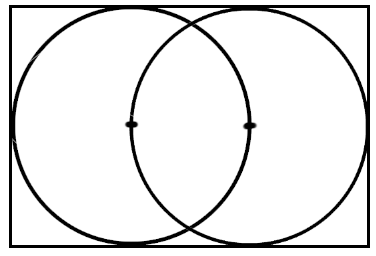# Two Congruent Circles

Geometry Level 2The above diagram shows 2 congruent circles that are enclosed within a rectangle. Each circle passes through the center of the other circle. Each circle has an area of 10 units.

To the nearest whole number, what is the area of the rectangle?

Details & Assumptions:

1) Area of a circle$=\Pi { r }^{ 2 }$.

2) The two circles have the same area.

×